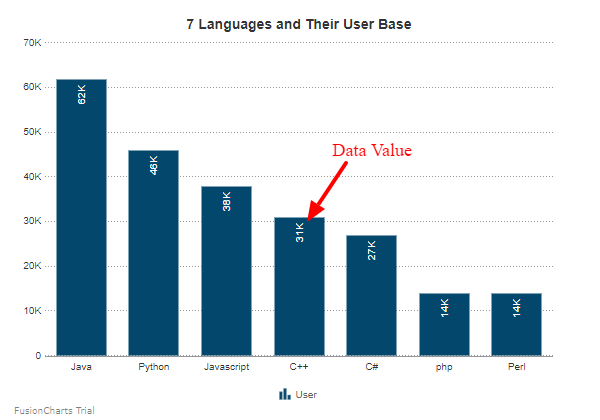# Data Value

Data values are plot values, i.e. values of each data plot (line, column, bar, pie) displayed on the chart.## Customize Data Value

In FusionCharts.NET, you can customize data values in the following ways with the help of different instance methods of the `Values` object (which accepts a string argument) under the `visualizationObj` object:

### Show/Hide Data Value

To display the data labels, set the value of the `Show` property to `true`. Refer to the code given below:

``visualizationObj.Values.Show = true;``

The chart will look as shown below:

FusionCharts will load here..

### Set the Limits

To hide the limit of the chart, set the value of `ShowLimits` property to `false`. Refer to the code given below:

``visualizationObj.Values.ShowLimits = false;``

The chart will look as shown below:

FusionCharts will load here..

### Rotate Values

To rotate the data values of the chart, set the value of `Rotate` property to `true`. Refer to the code given below:

``visualizationObj.Values.Rotate = true;``

The chart will look as shown below:

FusionCharts will load here..

### Data Value Position

If you've opted to show data values on the chart, `ValuePosition` property lets you adjust the vertical alignment of data values with respect to dataplots. By default, this attribute is set to AUTO mode in which the alignment of each data value is determined automatically based on the position of each plot point. In ABOVE mode, data values are displayed above the plot points unless a plot point is too close to the upper edge of the canvas while in BELOW mode, data values are displayed below the plot points unless a plot point is too close to the lower edge of the canvas.

Refer to the code given below:

``visualizationObj.Values.Position = ValueFormat.ValuePosition.AUTO;``

### Highlight Effect

`PlotHighlightEffect` property lets you enable/disable the highlight effect, in the form of a `fadeout`, for the data plots corresponding to a legend item. When this attribute is enabled and the mouse pointer is hovered over a legend item label, plots belonging to the other data series fadeout, by reducing their opacity.

The fadeout effect can be customized by specifying the fadeout color and opacity using the `color` and `alpha` attributes. Eg. `fadeout|color=#ff0000, alpha=60`

Refer to the code below:

``visualizationObj.Values.PlotHighlightEffect = "fadeout|color=#ff0000, alpha=60";``

### Place Value

To show the data values inside the column, set the `PlaceValueInside` property to `true`. Refer to the code given below:

``visualizationObj.Values.PlaceValueInside =true;``

The chart looks like as shown below:

FusionCharts will load here..